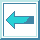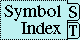#Macro PROG, PROG*

Syntax:

prog ({var | (var [init-form])}*) declaration* {tag | statement}*

=> result*

prog* ({var | (var [init-form])}*) declaration* {tag | statement}*

=> result*

Arguments and Values:

var---variable name.

init-form---a form.

declaration---a declare expression; not evaluated.

tag---a go tag; not evaluated.

statement---a compound form; evaluated as described below.

results---nil if a normal return occurs, or else, if an explicit return occurs, the values that were transferred.

Description:

Three distinct operations are performed by prog and prog*: they bind local variables, they permit use of the return statement, and they permit use of the go statement. A typical prog looks like this:

``` (prog (var1 var2 (var3 init-form-3) var4 (var5 init-form-5))
declaration*
statement1
tag1
statement2
statement3
statement4
tag2
statement5
...
)
```

For prog, init-forms are evaluated first, in the order in which they are supplied. The vars are then bound to the corresponding values in parallel. If no init-form is supplied for a given var, that var is bound to nil.

The body of prog is executed as if it were a tagbody form; the go statement can be used to transfer control to a tag. Tags label statements.

prog implicitly establishes a block named nil around the entire prog form, so that return can be used at any time to exit from the prog form.

The difference between prog* and prog is that in prog* the binding and initialization of the vars is done sequentially, so that the init-form for each one can use the values of previous ones.

Examples:

```(prog* ((y z) (x (car y)))
(return x))
```
returns the car of the value of z.

``` (setq a 1) =>  1
(prog ((a 2) (b a)) (return (if (= a b) '= '/=))) =>  /=
(prog* ((a 2) (b a)) (return (if (= a b) '= '/=))) =>  =
(prog () 'no-return-value) =>  NIL
```
``` (defun king-of-confusion (w)
"Take a cons of two lists and make a list of conses.
Think of this function as being like a zipper."
(prog (x y z)          ;Initialize x, y, z to NIL
(setq y (car w) z (cdr w))
loop
(cond ((null y) (return x))
((null z) (go err)))
rejoin
(setq x (cons (cons (car y) (car z)) x))
(setq y (cdr y) z (cdr z))
(go loop)
err
(cerror "Will self-pair extraneous items"
"Mismatch - gleep!  ~S" y)
(setq z y)
(go rejoin))) =>  KING-OF-CONFUSION
```
This can be accomplished more perspicuously as follows:

``` (defun prince-of-clarity (w)
"Take a cons of two lists and make a list of conses.
Think of this function as being like a zipper."
(do ((y (car w) (cdr y))
(z (cdr w) (cdr z))
(x '() (cons (cons (car y) (car z)) x)))
((null y) x)
(when (null z)
(cerror "Will self-pair extraneous items"
"Mismatch - gleep!  ~S" y)
(setq z y)))) =>  PRINCE-OF-CLARITY
```

Affected By: None.

Exceptional Situations: None.

Notes:

prog can be explained in terms of block, let, and tagbody as follows:

``` (prog variable-list declaration . body)
==  (block nil (let variable-list declaration (tagbody . body)))
```

The following X3J13 cleanup issue, not part of the specification, applies to this section: